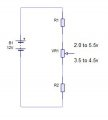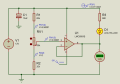# Calculating the value of resistors to obtain a range of voltages

Thread Starter

#### Ford Prefect

Joined Jun 14, 2010
201
Does anyone know of an easy way of calculating the value of resistors (R1,VR1,R2 in the photo) to obtain a range of voltages.
In other words... I have a voltage source of 12vDC and want to calculate the resistor values to obtain output voltages at the wiper of a potentiometer of:

1. voltage output of between 2.0 volts and 5.5 volts

(2.0 volts when the potentiometer is close to lowest setting / 5.5 volts when the potentiometer is close to highest setting).
2. voltage output of between 3.5 volts and 4.5 volts
(3.5 volts when the potentiometer is close to lowest setting / 4.5 volts when the potentiometer is close to highest setting).I want the potentiometer to be as sensitive as possible to obtain 1/10th volt between the ranges. Eg. as in 1 above..2.0v, 2.1v, 2.2v, 2.3v etc.

Last edited:
Thread Starter

#### Ford Prefect

Joined Jun 14, 2010
201
hi FP.
To ensure that any load connected to the wiper does not effect the range/accuracy, you need a HiZ buffer OPA on the wiper output.
I would suggest you consider a multi-turn pot with a slow motion drive /dial
Look at this link.
http://www.resistorguide.com/potentiometer/
E
Update: Dial Knobs
https://www.google.com/search?q=mul...fAhV5ThUIHa90AqoQsAR6BAgEEAE&biw=1388&bih=896
Hello again Eric..and thanks.
But do you know of an easy way of calculating the value of the resistors?
Is there an online calculator or application I can use?

#### ericgibbs

Joined Jan 29, 2010
12,976
hi FP,
Not seen an online calc, will have a look around.
Remember the pot resistance will have to be a preferred value, eg: 1K 5K 10K etc... this will decide the R1 and R2 values
E
This image and LTS shows one way of checking your results.E

Update:
Added example for the second range

#### Attachments

• 28 KB Views: 5
• 674 bytes Views: 1
• 24 KB Views: 3
• 674 bytes Views: 1
Last edited:
•Ford Prefect
Thread Starter

#### Ford Prefect

Joined Jun 14, 2010
201
hi FP,
Not seen an online calc, will have a look around.
Remember the pot resistance will have to be a preferred value, eg: 1K 5K 10K etc... this will decide the R1 and R2 values
E
Yes, thanks again Eric, I am aware of the pot resistance will decide the value of R1 and R2 and I have been juggling about with different resistor values trying to find the outputs I want but it's long and tiresome. Maybe I can write and Excel macro to change R1 and R2 by a few ohms each step and fix VR1 to try to get the outputs.
It is also possible to make VR1 as 1k and with a 1k (or other) fixed resistor in parallel.

#### ericgibbs

Joined Jan 29, 2010
12,976
hi,
You have shown only two voltage range circuits in your opening post are there more.?
To solve just for two options wouldn't justify writing an Excel routine.
I have updated post #4 showing the second circuit.
I chose a 10K pot in order to reduce the current loading on the 12V supply. R1 and R2 can be higher values.
E

#### ericgibbs

Joined Jan 29, 2010
12,976
hi,
These are the results for a 1K pot, just divide the R1/R2 values by 10.
E

#### absf

Joined Dec 29, 2010
1,949
This is the way I would do it without much tedious calculation....Lets use problem 1 as an example.
Since you need 2.0 to 5.5V and your supply is 12V. So I use 10KΩ/V as the basis of calculation.

So the circuit would turn up as in the circuit above.
And you wouldn't be able to get 35K pot of course, so lets use 100K pot just as an example. 100K/35K= 2.857.....
Use that factor as a constant and multiply R1 and R2 by the same factor and get the nearest value in E24 or 1% range resistors.

Allen

•Ford Prefect

#### ericgibbs

Joined Jan 29, 2010
12,976
hi.
A quick fix while I find the pot models.
Replace the 1k pot with a 1K resistor and measure top and bottom voltages of that resistor.
I will post the pot models.
E

Update:
Unzip this potzip, place the files in the same folder as the voltage divider you are developing.
Later on, move the .sub to the LTS folder SUB folder and the .asy to the SYM folder.

It includes a simple test asc

#### Attachments

• 1 KB Views: 3
Last edited:
•Ford Prefect
Thread Starter

#### Ford Prefect

Joined Jun 14, 2010
201
This is the way I would do it without much tedious calculation....

View attachment 165341
Lets use problem 1 as an example.
Since you need 2.0 to 5.5V and your supply is 12V. So I use 10KΩ/V as the basis of calculation.

So the circuit would turn up as in the circuit above.
And you wouldn't be able to get 35K pot of course, so lets use 100K pot just as an example. 100K/35K= 2.857.....
Use that factor as a constant and multiply R1 and R2 by the same factor and get the nearest value in E24 or 1% range resistors.

Allen
Actually Allen this is good.
I have used a 25k pot with 68k and 20k for the resistors and getting between 1.8v and 6.4v for the voltage outputs.
This should be a good basis to start.

Last edited:

#### bertus

Joined Apr 5, 2008
21,371
Hello,

When you are using a 25K pot instead of a 35K pot, multiply the values by (25/35).
The 20K will become 14.29K
the 68K will become 48.57K

Bertus

•Ford Prefect
Thread Starter

#### Ford Prefect

Joined Jun 14, 2010
201
Hello,
When you are using a 25K pot instead of a 35K pot, multiply the values by (25/35).
The 20K will become 14.29K
the 68K will become 48.57K
Bertus
Cool, thanks Bertus#### Jony130

Joined Feb 17, 2009
5,233
1. voltage outut of between 2.0 volts and 5.5 volts
(2.0 volts when the potentiometer is close to lowest setting / 5.5 volts when the potentiometer is close to highest setting).
The voltage across POT is:

Vpot = 5.5V - 2V = 3.5V

So if your pot is 25kΩ the pot current is Ipot = 3.5V/25kΩ = 140μA.

By knowing this we can solve for R1 and R2.

R1 = (12V - 5.5V)/140μA = 46.4kΩ

R2 = 2V/140μA = 14.29kΩ

•absf and Ford Prefect